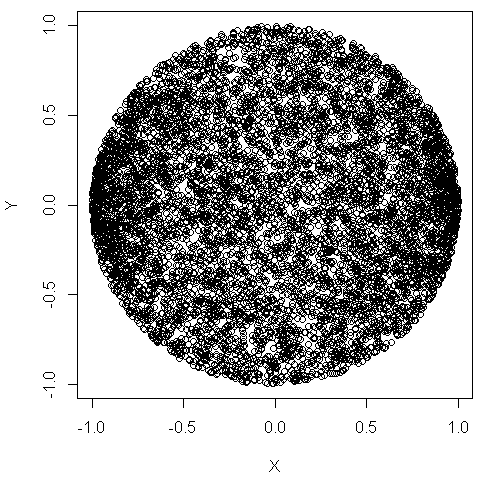# How to use Python script as external test data source?

The powerful pattern engine is a part of all test data generation software by DTM soft and provides a lot of related methods. However, it is not suitable for some cases because can't read or generate data directly. There are samples:

• Reading complex text or binary external file with test data
• Data that requires mathematical transformations
• Remote data sets without standard access over file system or HTTP/FTP request, etc.

Our data generation engine provides two solutions for this problem. By performance reason, the user can build own DLL using language like C++. The alternative is scripting language. The engine supports any installed at user's system language: Perl, Ruby Python, etc. Moreover, if node.js environment is installed Java Script can be used as well.

Due to internal design and performance reasons the engine calls script only once for each column of the data set to be generated. This design makes some limitation for script usage. In this article, we'll discuss optimal ways to use Python scripts as a data source for test data generation.

### Simple Script Call

In our simplest example, we just call a script. In our test system, the interpreter is located at E:\apps\Python34\python.exe and we'll store our scripts at d:\scripts folder. Well, our first call is
$Script(E:\apps\Python34\python.exe,D:\scripts\sqrts.py) Our script will calculate square roots of odd numbers between 1 and 256 those divisible by 3: import math for i in range(1,256): if (i % 7)==0 and (i % 2): print(math.sqrt(i))  As you can see the program just writes generated data to standard output stream. There are sample results shown by DTM Flat File Generator's preview:### Passing Parameter to the Script In our second example, we'll pass some value to the script. To make it more interesting let us pass constant value from another column (named F2) as parameter's value. There is call:$Script(E:\apps\Python34\python.exe,D:\scripts\sqrts2.py,@'F2')

The script shifts output value to passed parameter:

import math
import sys

for i in range(1,256):
if (i % 7)==0 and (i % 2):
print(math.sqrt(i)+float(sys.argv))


### Group Based on Script Call Results

In this example, we'll prepare a set of the pair (X,Y) coordinates in a circle with radius 1 around (0,0). For this purpose, we have to use $ScriptGroup function instead of$Script. We should create a group of two columns X and Y.

Our script is

import math
import random

for i in range(1,5000):
x = random.uniform(-1,1)
yMax = math.sqrt(1-x*x)
y = random.uniform(-yMax,yMax)
print(x,"\t",y)


As you can see the script creates 5000 dots and writes them to tab-delimited stream. In the data generator we can access to the x coordinate by the following call:
$ScriptGroup(1,1,E:\apps\Python34\python.exe,D:\scripts\in-circle.py) The second column can be accessed by$Group(1,2) engine call

The results of the software execution we can see at the plot: